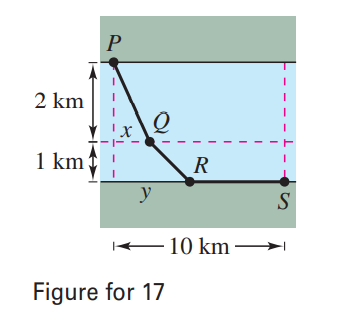×
Get Full Access to Calculus: Early Transcendental Functions - 6 Edition - Chapter 13.9 - Problem 17
Get Full Access to Calculus: Early Transcendental Functions - 6 Edition - Chapter 13.9 - Problem 17

×

# ?A water line is to be built from point P to point S and must pass through regions where construction costs differ (see figure). The cost per kilometerISBN: 9781285774770 141

## Solution for problem 17 Chapter 13.9

Calculus: Early Transcendental Functions | 6th Edition

• Textbook Solutions
• 2901 Step-by-step solutions solved by professors and subject experts
• Get 24/7 help from StudySoup virtual teaching assistantsCalculus: Early Transcendental Functions | 6th Edition

4 5 1 235 Reviews
25
0
Problem 17

A water line is to be built from point P to point S and must pass through regions where construction costs differ (see figure). The cost per kilometer (in dollars) is 3k from P to Q, 2k from Q to R, and k from R to S. Find x and y such that the total cost C will be minimized.Step-by-Step Solution:

Step 1 of 5) in a given order. Each of a1, a2, a3 and so on represents a number. These are the terms of the sequence. For example, the sequence

Step 2 of 2

## Discover and learn what students are asking

Statistics: Informed Decisions Using Data : The Randomized Complete Block Design
?Given the following ANOVA output, answer the questions that follow: (a) The researcher wants to test H0: m1 = m2 = m3 =

Statistics: Informed Decisions Using Data : Testing the Significance of the Least-Squares Regression Model
?What do the y-coordinates on the least-squares regression line represent?

Unlock Textbook Solution